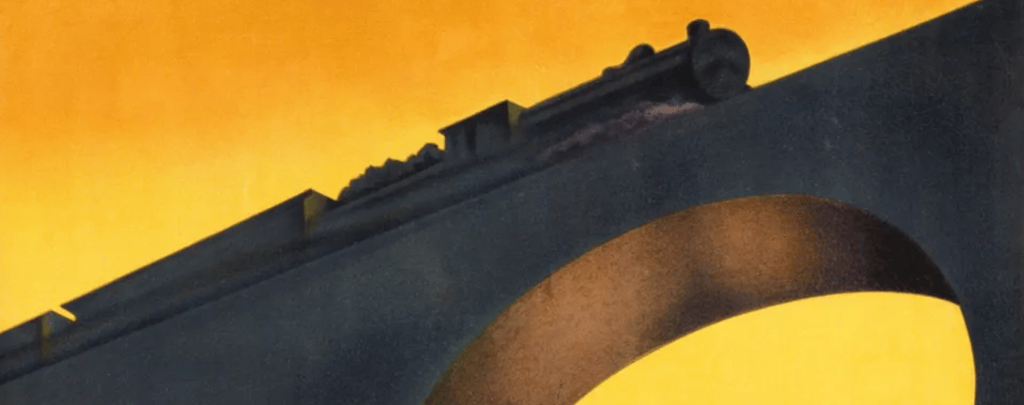# Anaglyphs

Anaglyph is just a fancy word for the 3D stereoscopics effect achieved by offsetting and blending a red and cyan tinted version of an image.To view such an image and observe the so called 3D effect, naturally one will need to have glasses with red an cyan colored lenses at hand.

As per usual, I went ahead and created a small command line utility that can process an input image and spit out its anaglyph counterpart.

Let’s take awe a gander at how this is done by taking a look at the code snippet below.

``````#define clamp(x, max) ((x) > (max) ? (max) : (x))

static void process(
const rgba_t *restrict input,
rgba_t *restrict output,
const int w,
const int h,
const int dx
)
{
int x, y, yo, i, ww;
rgba_t c, a;

ww = w - 1;

for(y = 0; y < h; y++)
{
yo = y * w;
for(x = 0; x < w; x++)
{
i = x + yo;
c = input[i];

a = input[clamp(x + dx, ww) + yo];
c.r = a.r;

output[i] = c;
}
}
}
``````

We simply iterate over every pixel and sample ahead `dx` amount of pixels on the `x` axis, while also making sure that `x` stays within the bounds of the image.

Then, we replace the `red` channel of the current pixel with the `red` channel of the pixel we sampled, while leaving the `green` and `blue` channels untouched.

And now, drum rolls please! You can admire the results in all its glory. Slide over the image to view the difference. Special thanks go out to Big Buck Bunny for participating and being a trusty test subject.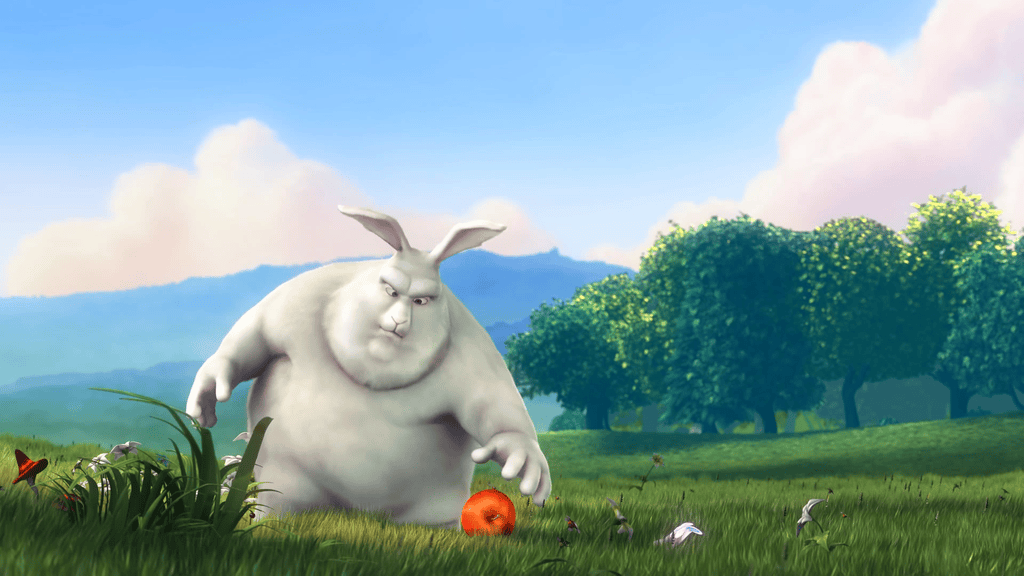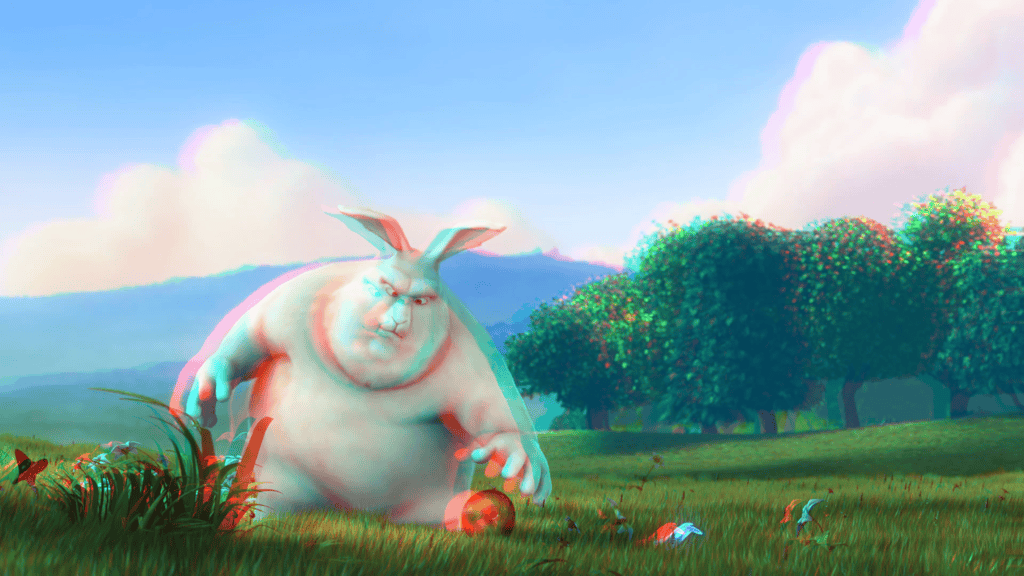If you pay attention and take a closer look to the right side of the image, you might notice how there are some artifacts that look quite bad to say the least.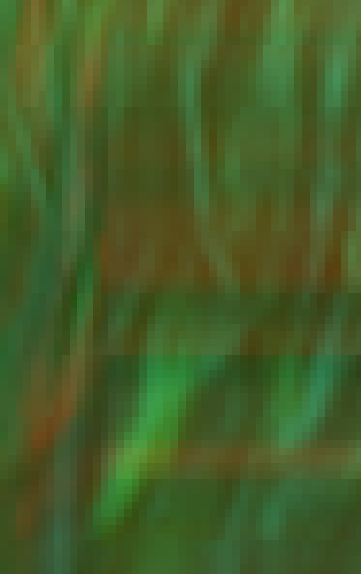These are caused by the fact that we clamp `x` to the range of `0 .. w - 1`. With some images this might be less or barely noticeable, but still ugly and not proper at all.

Nonetheless, an easy way to get rid this issue would be to simply crop the output image. Presto, problem solved. But, there’s another way we can mitigate this without having to resort to something as drastic as cropping.

We simply never sample beyond the bounds, namely `w - dx` and then we simply copy the rest of the pixels from the input image as-is.

``````static void process(
const rgba_t *restrict input,
rgba_t *restrict output,
const int w,
const int h,
const int dx
)
{
int x, y, yo, i, ww;
rgba_t c, a;

ww = w - dx;

for(y = 0; y < h; y++)
{
yo = y * w;

for(x = 0; x < ww; x++)
{
i = x + yo;
c = input[i];

a = input[i + dx];
c.r = a.r;

output[i] = c;
}

for(x = ww; x < w; x++)
{
i = x + yo;
output[i] = input[i];
}
}
}
``````

While this is most definitely far from being perfect, it’s also not terrible. At any rate much better than the ugly artifacts and also a whole lot less noticeable, which is a plus.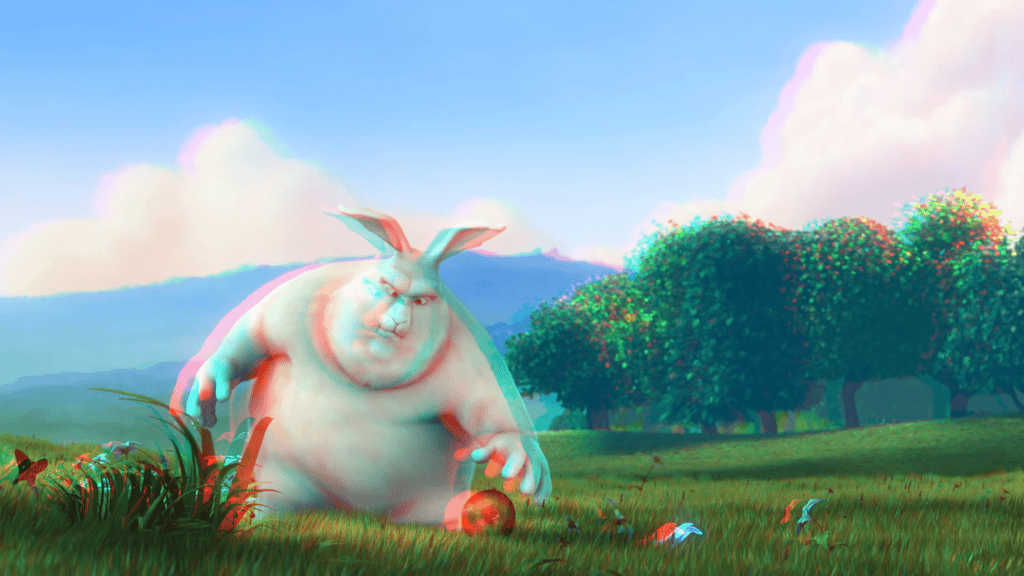Before I go much further, let’s take a look at what happens if we flip the channels around.

``````static void process(
const rgba_t *restrict input,
rgba_t *restrict output,
const int w,
const int h,
const int dx
)
{
int x, y, yo, i, ww;
rgba_t c, a;

ww = w - dx;

for(y = 0; y < h; y++)
{
yo = y * w;

for(x = 0; x < ww; x++)
{
i = x + yo;
c = input[i];

a = input[i + dx];
c.g = a.g;
c.b = a.b;

output[i] = c;
}

for(x = ww; x < w; x++)
{
i = x + yo;
output[i] = input[i];
}
}
}
``````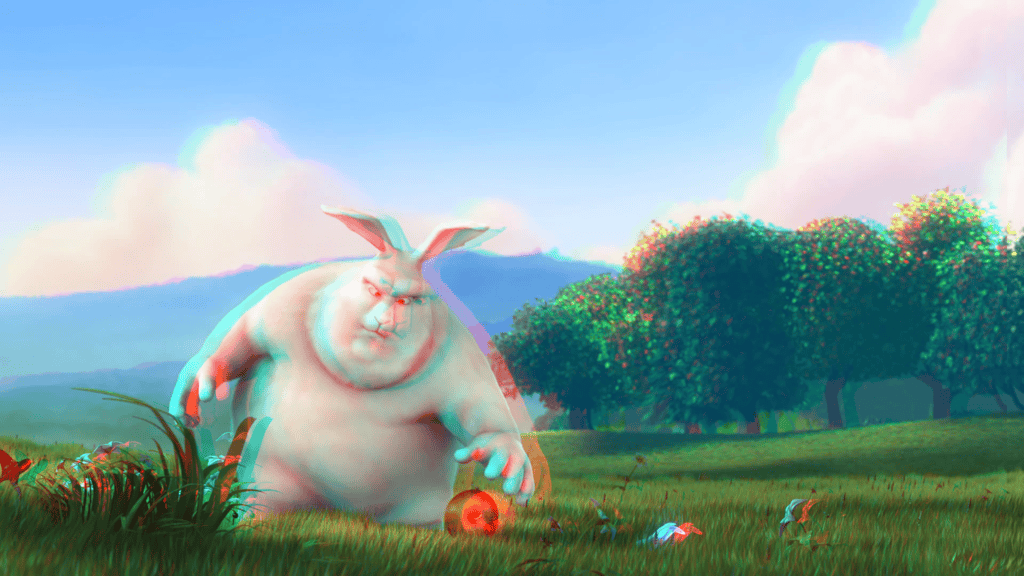To observe the difference a wee bit better, let’s take a look another look at the two versions overlay-ed on top of each other.In case you want to play around with this yourself, you can find the full listing of the utility below.

``````/*
*/
#include <stdbool.h>
#include <stdint.h>
#include <stdlib.h>
#include <stdio.h>
#include <math.h>

#define STBI_NO_HDR
#define STBI_NO_LINEAR
#define STB_IMAGE_IMPLEMENTATION
#define STB_IMAGE_WRITE_IMPLEMENTATION
#include "stb_image.h"
#include "stb_image_write.h"

#ifndef UNUSED
#define UNUSED(x) (void)(x)
#endif

#ifndef DEFAULT_X_OFFSET
#define DEFAULT_X_OFFSET 32
#endif

typedef union
{
uint32_t rgba;
struct
{
uint32_t r : 8;
uint32_t g : 8;
uint32_t b : 8;
uint32_t a : 8;
};
} rgba_t;

static void process_red(
const rgba_t *restrict input,
rgba_t *restrict output,
const int w,
const int h,
const int dx
);
static void process_cyan(
const rgba_t *restrict input,
rgba_t *restrict output,
const int w,
const int h,
const int dx
);

int main(int argc, char *argv[])
{
int w, h, dx, bpp, ret;
bool flip;
rgba_t *input, *output;

if(argc < 3)
{
fprintf(stderr, "usage: %s input.png output.png [dx]\n", argv);
return EXIT_FAILURE;
}

if(argc > 3)
{
dx = atoi(argv);
}
else
{
dx = DEFAULT_X_OFFSET;
}

flip = dx < 0;

if(flip)
{
dx = -dx;
}

input = (rgba_t *) stbi_load(argv, &w, &h, &bpp, sizeof(rgba_t));
if(input == NULL)
{
fprintf(
stderr,
stbi_failure_reason()
);
return EXIT_FAILURE;
}

output = malloc(w * h * sizeof(rgba_t));
if(output == NULL)
{
fprintf(stderr, "failed to allocate memory for output image\n");
stbi_image_free(input);
return EXIT_FAILURE;
}

if(flip)
process_cyan(input, output, w, h, dx);
else
process_red(input, output, w, h, dx);

ret = stbi_write_png(
argv,
w,
h,
sizeof(rgba_t),
output,
w * sizeof(rgba_t)
);
if(ret == 0)
{
fprintf(stderr, "failed to write output image: '%s'\n", argv);
ret = EXIT_FAILURE;
}
else
{
fprintf(stdout, "wrote output image to '%s' ...\n", argv);
ret = EXIT_SUCCESS;
}

free(output);
stbi_image_free(input);
return ret;
}

static void process_red(
const rgba_t *restrict input,
rgba_t *restrict output,
const int w,
const int h,
const int dx
)
{
int x, y, yo, i, ww;
rgba_t c, a;

ww = w - dx;

for(y = 0; y < h; y++)
{
yo = y * w;

for(x = 0; x < ww; x++)
{
i = x + yo;
c = input[i];

a = input[i + dx];
c.r = a.r;

output[i] = c;
}

for(x = ww; x < w; x++)
{
i = x + yo;
output[i] = input[i];
}
}
}

static void process_cyan(
const rgba_t *restrict input,
rgba_t *restrict output,
const int w,
const int h,
const int dx
)
{
int x, y, yo, i, ww;
rgba_t c, a;

ww = w - dx;

for(y = 0; y < h; y++)
{
yo = y * w;

for(x = 0; x < ww; x++)
{
i = x + yo;
c = input[i];

a = input[i + dx];
c.g = a.g;
c.b = a.b;

output[i] = c;
}

for(x = ww; x < w; x++)
{
i = x + yo;
output[i] = input[i];
}
}
}

/* vim: set ts=4 sw=4 sts=4 noet: */
``````

Damn, I nearly dated myself there for a moment. Oh well, at least I didn’t say subroutine.

Naturally, not all images will lend themselves well to this technique and more than likely the offset will have to be tweaked on a per image basis in order to achieve even remotely half decent results.

After taking a peek at the code above, I am sure that it might have occurred to you, if this could be applied in real time as a filter when playing video or a video game?

The answer to that question is a resounding yes. Ancient Astronaut theorists also seem to agree and suggest that further clues can be found by examining the fragment shader recently discovered in the stepped pyramid which served as the tomb and resting place of Kukulkan.

``````#define SHADER_SOURCE(...) #__VA_ARGS__

uniform sampler2D texture;
uniform vec3 offset;
varying vec2 uv;

void main()
{
vec4 a = texture2D(texture, uv);
vec4 b = texture2D(texture, uv + offset.xy);

float s = step(uv.x, offset.z);
gl_FragColor = mix(a, vec4(a.r, b.g, b.b, b.a), s);
}
);
``````

It assumes that we are rendering a full screen quad using a `2D sampler` with its `UV` coordinate wrapping set to `GL_CLAMP_TO_EDGE`.

The value of the offset uniform would be something like:

``````vec3(0.01, 0.0, 1.0 - 0.01);
``````

In the context of a game, you’d render into two separate render targets and then sample and mix the channels together in a very simple and dumb fragment shader. This would probably give you the best bang for your buck.

``````#define SHADER_SOURCE(...) #__VA_ARGS__

uniform sampler2D texture_leye;
uniform sampler2D texture_reye;
varying vec2 uv;

void main()
{
vec4 a = texture2D(texture_leye, uv);
vec4 b = texture2D(texture_reye, uv);

gl_FragColor = vec4(a.r, b.g, b.b, b.a);
}
);
``````

Alternatively, you could avoid using render targets, and render twice with additive blending. This can also be done with just fixed function pipeline without the need for any shaders or multi-texturing for that matter. Perfect for the scenario when you’d really want to bring grandma’s PC stuck in the attic alive with only a few lines of code.

It should go without saying that this is the most basic way of constructing anaglyphs and there are several more advanced variations and approaches out there in the great unknown. So please, don’t consider this as some authoritative technique and reference implementation.

Personally, I always wondered how come stereoscopy as a whole has never seen a more widespread adoption, especially in more recent years.

But then again, VR is also struggling, and what these two have in common? The need for an additional accessory, in other words a pair of special glasses.

As it turns out, people really seem to be in a love hate relationship glasses, so much so, that this innate hate spawned an entire industry. I am talking about contact lenses of course.

2023-07-08